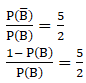There are three events A, B, C one of which must and only one can happen, the odds are 8 to 3 against A, 5 to 2 against B, fins the odds against C.

Asked by Aaryan | 1 year ago |  32

##### Solution :-

As, out of 3 events A, B and C only one can happen at a time which means no event have anything common.

We can say that A, B and C are mutually exclusive events.

So, by definition of mutually exclusive events we know that:

P (A ∪ B ∪ C) = P (A) + P (B) + P (C)

According to question one event must happen.

So, A or B or C is a sure event.

P (A ∪ B ∪ C) = 1 … Equation (1)

We need to find odd against C:

Given:

Odd against A = $$\dfrac{8}{3}$$

8 P (A) = 3 – 3 P (A)

11 P (A) = 3

P (A) = $$\dfrac{3}{11}$$ … Equation (2)

Similarly, we are given with: Odd against B = $$\dfrac{5}{2}$$5 P (B) = 2 – 2 P(B)

7 P (B) = 2

P (B) =$$\dfrac{2}{7}$$ …Equation (3)

From equation 1, 2 and 3 we get:

P (C) = 1 – $$\dfrac{3}{11}$$ – $$\dfrac{2}{7}$$

= $$\dfrac{ (77-21-22)}{77}$$

$$\dfrac{34}{77}$$

So, P (C′) = 1 – ($$\dfrac{34}{77}$$)

=$$\dfrac{43}{77}$$

Odd against C:

$$\dfrac{43}{34}$$

Answered by Aaryan | 1 year ago

### Related Questions

#### One number is chosen from numbers 1 to 100. Find the probability that it is divisible by 4 or 6?

One number is chosen from numbers 1 to 100. Find the probability that it is divisible by 4 or 6?

#### The probability that a student will pass the final examination in both English and Hindi is 0.5

The probability that a student will pass the final examination in both English and Hindi is 0.5 and the probability of passing neither is 0.1. If the probability of passing the English examination is 0.75. What is the probability of passing the Hindi examination?

#### A card is drawn from a deck of 52 cards. Find the probability of getting an ace or a spade card.

A card is drawn from a deck of 52 cards. Find the probability of getting an ace or a spade card.